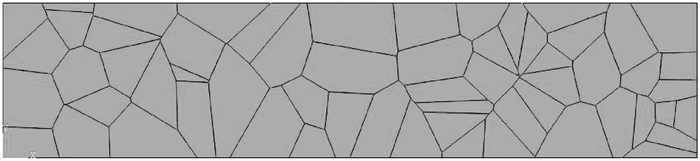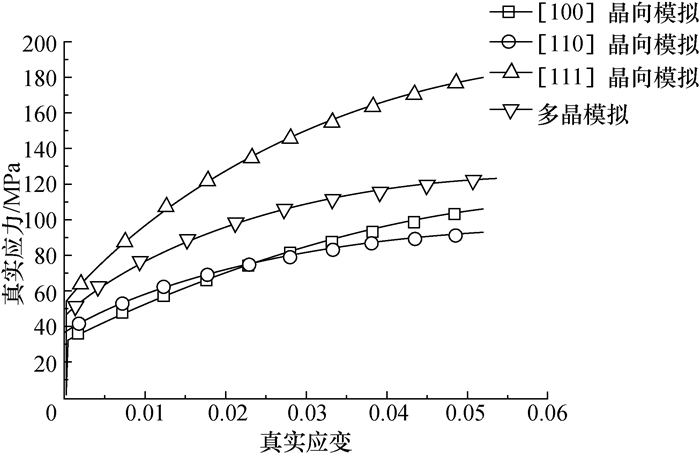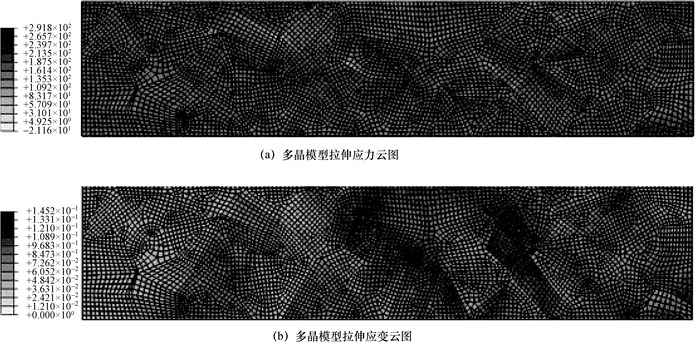, 汤镇睿 , 张海泉 , 李红克 , 王鑫

Crystal plasticity constitutive model for BCC based on the dislocation density
, TANG Zhenrui, ZHANG Haiquan, LI Hongke, WANG Xin
Institute of Nuclear and New Energy Technology, Tsinghua University, Beijing 100084, China
Abstract: Crystal plasticity theory is a fundamental theory that combines the crystal microscopic slip mechanism with macroscopic plastic deformation to predict meso-scale plastic deformation. The dislocation density has an important influence on the hardening behavior of metal crystals. This paper presents a constitutive model based on crystal plasticity theory and dislocation motion theory for the BCC crystal structure. The model is used to study the mechanical behavior of a BCC lattice. Using the UMAT subroutine in ABAQUS for numerical simulations of a uniaxial tensile tests of single crystal and polycrystal iron. The results show that the constitutive model effectively simulates the mechanical behavior of the uniaxial tensile test for single crystal and polycrystal iron.
Key words: crystal plasticity     dislocation motion     body center cubic (BCC)     uniaxial tensile     finite element method (FEM)

1 基于位错运动的BCC晶体塑性本构模型 1.1 晶体塑性理论

 $F = {F^ * }{F^P}.$ (1)

L表示速度梯度张量，将其分解为弹性变形部分L*和塑性变形部分Lp

 $L = \dot F{F^{ - 1}} = {L^ * } + {L^P},$ (2)
 ${L^ * } = {{\dot F}^ * }{F^{ * - 1}},$ (3)
 ${L^P} = {{\dot F}^p}{F^{p - 1}} = \sum\limits_{a = 1}^n {{{\dot \gamma }^\alpha }{s^{ * \alpha }} \otimes {m^{ * \alpha }}} .$ (4)

 ${\tau ^\alpha } = \tau :{P^\alpha }.$ (5)

 ${P^\alpha } = \frac{{{s^\alpha }{m^\alpha } + {m^\alpha }{s^\alpha }}}{2}.$
1.2 位错滑移运动

 $\left\{ \begin{array}{l} {{\dot \gamma }^\alpha } = 0,\;\;\;\;\;\;\;\;\;\;\;\;\;\;\;\;\;\;\;\;\;\;\;\;\;\;\;\;\;\;\;\;\;\;\;\;\;\;\;\;\;\;\;\;\;\;\;\;\;\;\;\;\;\;\;\;\;\;\;\;\;\;\;\;\;\;\;\;\;\left| {{\tau ^\alpha }} \right| < {g^\alpha };\\ {{\dot \gamma }^\alpha } = \dot \gamma _0^\alpha \exp \left\{ { - \frac{{{Q_0}}}{{kT}}{{\left[ {1 - {{\left( {\frac{{\left| {{\tau ^\alpha }} \right| - {g^\alpha }}}{{{{\hat \tau }^\alpha }}}} \right)}^p}} \right]}^q}} \right\}{\mathop{\rm sgn}} \left( {{\tau ^\alpha }} \right),\;\;\;\;\;\;\;\;\;\;\;\left| {{\tau ^\alpha }} \right| > {g^\alpha }. \end{array} \right.$ (6)
 ${{\hat \tau }^\alpha } = \hat \tau _0^\alpha \frac{G}{{{G_0}}}.$ (7)

 ${g^\alpha } = Gb\sqrt {{q_\rho }\sum\limits_{\beta + 1}^N {\left[ {{A^{\alpha \beta }}\left( {\rho _{\rm{M}}^\beta + \rho _{\rm{I}}^\beta } \right)} \right]} } .$ (8)

 $\dot \rho _{\rm{M}}^\alpha = \left( {\frac{{{k_{{\rm{mul}}}}}}{{b{l_{\rm{d}}}}} - \frac{{2{R_{\rm{c}}}}}{b}\rho _{\rm{M}}^\alpha - \frac{1}{{b{\lambda ^\alpha }}}} \right)\left| {{{\dot \gamma }^\alpha }} \right|,$ (9)
 $\dot \rho _{\rm{I}}^\alpha = \left( {\frac{1}{{b{\lambda ^\alpha }}} - {k_{{\rm{dyn}}}}\rho _{\rm{I}}^\alpha } \right)\left| {{{\dot \gamma }^\alpha }} \right|.$ (10)

2 材料参数确定

3 计算结果与分析 3.1 单晶单轴拉伸实验模拟图 1 晶向计算与实验单轴拉伸应力-应变曲线

3.2 多晶单轴拉伸模拟图 2 多晶单轴拉伸模型

 $\left\{ \begin{array}{l} \bar \sigma = \sum\limits_{k = 1}^N {{v^k}{\sigma ^k}} ,\\ \bar \varepsilon = \sum\limits_{k = 1}^N {{v^k}{\varepsilon ^k}} . \end{array} \right.$ (11)图 3 多晶与单晶模型单轴拉伸应力-应变曲线图 4 多晶模型单轴拉伸得到的应力云图及应变云图

4 结论

  Hill R. Generalized constitutive relations for incremental deformation of metal crystals by multislip[J]. Journal of the Mechanics and Physics of Solids, 1966, 14(2): 95–102. DOI:10.1016/0022-5096(66)90040-8  Hill R. The essential structure of constitutive laws for metal composites and polycrystals[J]. Journal of the Mechanics and Physics of Solids, 1967, 15(2): 79–95. DOI:10.1016/0022-5096(67)90018-X  Hill R, Rice J R. Constitutive analysis of elastic-plastic crystals at arbitrary strain[J]. Journal of the Mechanics and Physics of Solids, 1972, 20(6): 401–413. DOI:10.1016/0022-5096(72)90017-8  Asaro R J, Rice J R. Strain localization in ductile single crystals[J]. Journal of the Mechanics and Physics of Solids, 1977, 25(5): 309–338. DOI:10.1016/0022-5096(77)90001-1  Asaro R J. Micromechanics of crystals and polycrystals[J]. Advances in Applied Mechanics, 1983, 23(8): 1–115.  Peirce D, Shih C F, Needleman A. A tangent modulus method for rate dependent solids[J]. Computers & Structures, 1984, 18(5): 875–887.  Clough R W. The finite element method in plane stress analysis[C]//Proceedings of the 2nd ASCE Conference on Electronic Computation. Pittsburgh, USA: ASCE, 1960.  王自强, 段祝平. 塑性细观力学[M]. 北京: 北京大学出版社, 1995. WANG Ziqiang, DUAN Zhuping. Plastic Meso Mechanics[M]. Beijing: Peking University Press, 1995. (in Chinese)  Busso E P. Cyclic Deformation of Monocrystalline Nickel Aluminide and High Temperature Coatings[D]. Cambridge: Massachusetts Institute of Technology, 2005.  Keh A S. Work hardening and deformation sub-structure in iron single crystals deformed in tension at 298 K[J]. Philosophical Magazine, 1965, 12: 9–30. DOI:10.1080/14786436508224942  Suzuki T, Koizumi H, Kirchner H O K. Plastic flow stress of b.c.c. transition metals and the Peierls potential[J]. Acta Metallurgica Et Materialia, 1995, 43(6): 2177–2187. DOI:10.1016/0956-7151(94)00451-X  Johnson R A, Oh D J. Analytic embedded atom method model for BCC metals[J]. Journal of Materials Research, 1989, 4(5): 1195–1201. DOI:10.1557/JMR.1989.1195  Brunner D, Diehl J. Strain-rate and temperature dependence of the tensile flow stress of high-purity α-iron above 250 K (regime I) studied by means of stress-relaxation tests[J]. Physica Status Solidi, 1991, 124(1): 155–170. DOI:10.1002/(ISSN)1521-396X  Spitzig W A, Keh A S. The role of internal and effective stresses in the plastic flow of iron single crystals[J]. Metallurgical & Materials Transactions B, 1970, 1(12): 3325–3331.  Kocks U F. Thermodynamics and Kinetics of Slip[M]. Oxford: Pergamon Press, 1975.  Hutchinson J W. Plastic stress-strain relations of F.C.C polycrystalline metals hardening according to Taylor's rule[J]. Journal of the Mechanics & Physics of Solids, 1964, 12(1): 11–24.